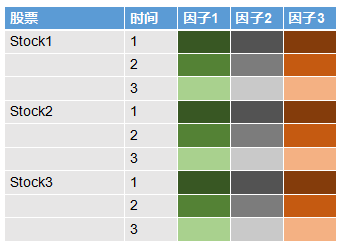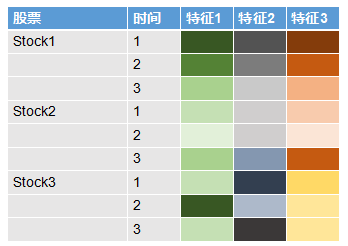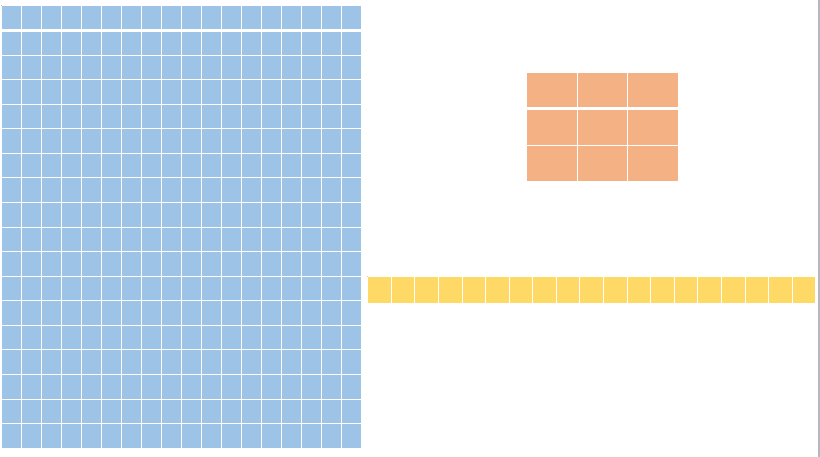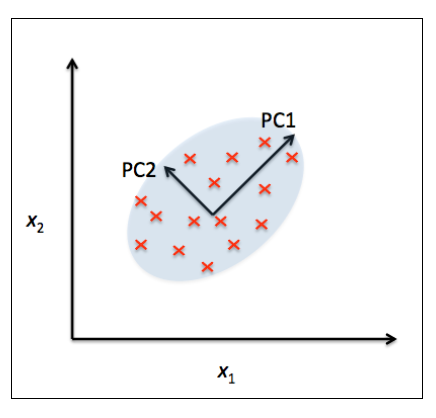(feynman0825) #1

# Alpha系列——因子模型¶

## 因子模型理论基础¶

#### 基本符号和概念¶

\begin{align*} x_{i,t} &= \alpha_i + \beta_{1,i} f_{1,t} + \beta_{2,i} f_{2,t} + \cdots + \beta_{k,i} f_{k,t} + \epsilon_{i,t}\\ &= \alpha_i + \boldsymbol{\beta^{\prime}_i \cdot f_t} + \epsilon_{i,t} \end{align*}

• $x_{i,t}$代表第i个资产在时间t的随机变量，如收益率
• $\alpha_i$代表资产i的截距
• $\boldsymbol{f_t}$表示共同因子，在不同的资产i之间保持常量
• $\boldsymbol{\beta}$表示资产i的因子负荷，或者可以理解为因子$\boldsymbol{f_t}$的参数向量
• $\epsilon_{i,t}$表示资产i的残差收益率，可以包含资产i的特异收益率\begin{align*} \boldsymbol{x_t} = \boldsymbol{\alpha} + B \cdot \boldsymbol{f_t} + \boldsymbol{\epsilon_{t}} \end{align*}

#### 模型基本假设¶

\begin{align*} \mathbb{E}[\boldsymbol{f_t}] &= \boldsymbol{\mu_f}\\ Cov[\boldsymbol{f_t}] &= \mathbb{E}[(\boldsymbol{f_t - \mu_f})(\boldsymbol{f_t - \mu_f})^{\prime}] = \Omega_f \end{align*}

\begin{align*} \mathbb{E}[\boldsymbol{\epsilon_t}] &= \boldsymbol{0_m}\\ Cov[\boldsymbol{\epsilon_t}] &= \mathbb{E}[\boldsymbol{\epsilon_t} \boldsymbol{\epsilon_t}^{\prime}] = \Psi\\ Cov[\boldsymbol{\epsilon_t},\boldsymbol{\epsilon_{t^{\prime}}}] &= \mathbb{E}[\boldsymbol{\epsilon_t} \boldsymbol{\epsilon_{t^{\prime}}}^{\prime}] = \boldsymbol{0} \quad \forall t \neq t^{\prime}\\ \end{align*}

\begin{align*} Cov[\boldsymbol{f_{i,t}},\boldsymbol{\epsilon_t}] &= \mathbb{E}[\boldsymbol{f_{i,t}} \boldsymbol{\epsilon_t^{\prime}}] = \boldsymbol{0}\\ \end{align*}

#### 模型基本性质¶

\begin{align*} \mathbb{E}[\boldsymbol{x_t} | \boldsymbol{f_t}] &= \boldsymbol{\alpha} + B \cdot \boldsymbol{f_t}\\ Cov[\boldsymbol{x_t} | \boldsymbol{f_t}] &= \Psi \end{align*}

\begin{align*} \mathbb{E}[\boldsymbol{x_t}] &= \boldsymbol{\alpha} + B \cdot \boldsymbol{\mu_t}\\ Cov[\boldsymbol{x_t}] &= B \Omega_f B^{\prime} + \Psi \end{align*}## 再论CAPM¶

CAPM把股票风险分解为系统风险和非系统风险：

\begin{align*} x_{i,t} = \alpha_i + \beta_i r_{m,t} + \epsilon_{i,t} \end{align*}

#### CAPM下的协方差矩阵¶

\begin{align*} \Sigma_x &= \boldsymbol{\beta \beta^T} \sigma_m^2 + diag(\sigma_1^2,\cdots ,\sigma_N^2)\\ &= \boldsymbol{\beta \beta^T} \sigma_m^2 + \Psi\\ &= \begin{pmatrix} \beta_1 \beta_1^T & \cdots & \beta_1 \beta_N^T \\ \vdots & \ddots & \vdots \\ \beta_N \beta_1^T & \cdots & \beta_N \beta_N^T \end{pmatrix} \sigma_m^2 + \begin{pmatrix} \sigma_1^2 & 0 & \boldsymbol{0} \\ 0 & \ddots & 0 \\ \boldsymbol{0} & 0 & \sigma_N^2 \end{pmatrix} \end{align*}

In :
start_date='2012-01-01'
end_date='2017-12-31'
instruments = ['601601.SHA', '600030.SHA', '601098.SHA', '600036.SHA', '000001.SZA']
index_col = '000906.SHA'
df = D.history_data(instruments+[index_col], start_date=start_date, end_date=end_date, fields=['close'])

# 重抽样还原至月度数据
resample_freq = 'M'
df_M = df.set_index('date')[['instrument','close']]\
.groupby('instrument')\
.apply(lambda df: df['close'].resample(resample_freq,how='last'))\
.transpose()\
.reset_index()\
.set_index('date')

# 得到收益率数据
ret_M = df_M.pct_change().dropna()

In :
from sklearn.linear_model import LinearRegression

X = ret_M[instruments]
r_m = ret_M[index_col]
reg = LinearRegression()
reg.fit(r_m.reshape(-1,1),X)
alpha = reg.intercept_
beta = reg.coef_
sigma2 = X.var()

In :
import matplotlib.pyplot as plt

plt.plot(beta,alpha,'o')
plt.title('alpha vs beta')
plt.xlabel('beta')
plt.ylabel('alpha')
plt.show()

In :
sigma2_m = r_m.var()
# 因子模型得到X协方差矩阵
Cov_X = np.outer(beta,beta)*sigma2_m + np.diag(sigma2)
Cov_X

Out:
array([[ 0.01381715,  0.00839504,  0.00701413,  0.00416245,  0.00381665],
[ 0.00839504,  0.04080852,  0.01255101,  0.00744824,  0.00682946],
[ 0.00701413,  0.01255101,  0.03183812,  0.00622308,  0.00570608],
[ 0.00416245,  0.00744824,  0.00622308,  0.01148666,  0.0033862 ],
[ 0.00381665,  0.00682946,  0.00570608,  0.0033862 ,  0.01222547]], dtype=float32)
In :
# 直接计算收益率协方差矩阵
X.cov()

Out:
instrument 601601.SHA 600030.SHA 601668.SHA 600036.SHA 601098.SHA
instrument
601601.SHA 0.009126 0.011894 0.008541 0.006183 0.002736
600030.SHA 0.011894 0.025787 0.018179 0.010236 0.006174
601668.SHA 0.008541 0.018179 0.021352 0.008945 0.004770
600036.SHA 0.006183 0.010236 0.008945 0.007794 0.002372
601098.SHA 0.002736 0.006174 0.004770 0.002372 0.009121

## Barra行业因子模型¶

#### 模型形式¶

Barra行业因子模型可以由如下形式表达：

\begin{align*} x_{i,t} = \beta_{i,1} f_{1,t} + \beta_{i,2} f_{2,t} + \cdots + \beta_{i,K} f_{K,t} + \epsilon_{i,t} \end{align*}

\begin{align*} \boldsymbol{x_t} = B \cdot \boldsymbol{f_t} + \boldsymbol{\epsilon_t} \end{align*}

$\beta_{i,k}$是事先知道的，$f_{k,t}$是不可观测的，是我们需要估计的参数，表示该行业因子在时间t的可实现收益率。另外x_{i,t}表示超额收益率，其他的模型假设和前面的因子模型相同。

#### 模型参数估计¶

\begin{align*} \boldsymbol{\beta_j^{\prime}} \boldsymbol{\beta_k} &= N_k \quad 如果 j=k\\ &= 0 \qquad 其他情况 \end{align*}

\begin{align*} \boldsymbol{\hat{f_t}} &= (B^{\prime}B)^{-1}B^{\prime} \boldsymbol{x_t}\\ &= \begin{pmatrix} \frac{1}{N_1} \sum_{i=1}^{N_1} x_{i,t} \mathbb{I}[\beta_{i,1}=1] \\ \vdots \\ \frac{1}{N_K} \sum_{i=1}^{N_K} x_{i,t} \mathbb{I}[\beta_{i,K}=K] \end{pmatrix} \end{align*}

\begin{align*} \hat{\Omega_f} &= \frac{1}{T-1} \sum_{t=1}^{T} (\boldsymbol{\hat{f_t} - \bar{f_t}})(\boldsymbol{\hat{f_t} - \bar{f_t}})^{\prime}\\ \boldsymbol{\bar{f_t}} &= \frac{1}{T} \sum_{t=1}^{T} \boldsymbol{\hat{f_t}} \end{align*}

\begin{align*} \hat{\Sigma_x} = B \hat{\Omega_f} B^{\prime} + \hat{\Psi} \end{align*}

#### 实验探索¶

In :
start_date='2012-01-01'
end_date='2018-12-31'
instruments = ['601601.SHA', '600030.SHA', '601098.SHA', '600036.SHA', '000001.SZA']
df = D.history_data(instruments, start_date=start_date, end_date=end_date, fields=['close','industry_sw_level1'])

# 重抽样还原至月度数据
resample_freq = 'M'
# 收益率矩阵
ret_M = df.set_index('date')[['instrument','close','industry_sw_level1']]\
.groupby('instrument')\
.apply(lambda df: df['close'].resample(resample_freq,how='last'))\
.transpose()\
.reset_index()\
.set_index('date')\
.pct_change()\
.dropna()
# 得到行业矩阵
industry_code = df.set_index('date')[['instrument','close','industry_sw_level1']]\
.groupby('instrument')\
.apply(lambda df: df['industry_sw_level1'].resample(resample_freq,how='last'))\
.transpose()\
.reset_index()\
.set_index('date')\
.drop_duplicates()\
.reset_index()\
.drop('date',axis=1)\
.stack()

In :
B = pd.get_dummies(industry_code,prefix='industry_').values
X = ret_M.values
F = np.linalg.inv(B.T.dot(B)).dot(B.T).dot(X.T)
Omega = np.cov(F,ddof=1)
errors = X-B.dot(F).T
Psi = errors.T.dot(errors)
Sigma = B.dot(Omega).dot(B.T) + Psi


## 统计因子模型¶

#### 主成分分析¶

\begin{align*} \boldsymbol{x_t} &= \alpha + B \cdot \boldsymbol{f_t} + \boldsymbol{\epsilon_t}\\ &= \alpha + \Gamma_k \boldsymbol{p_k} + \Gamma_{-k} \boldsymbol{p_{-k}} \end{align*}

\begin{align*} \Sigma_x &= P \Lambda P^{\prime}\\ PP^{\prime} &= \boldsymbol{I}\\ \Lambda &= diag(\lambda_1, \lambda_2, \cdots , \lambda_N) \quad \lambda_i从大到小排序 \end{align*}

P是我们的特征向量，$\Lambda$是我们的特征值。本质上PCA是一个降维技术，即从高维空间映射到它的子空间。如下图所示，我们可以通过子空间来最大限度得近似。\begin{align*} X^* &= UDV^{\prime}, X^*表示中心化后的X\\ \hat{\Lambda} &= \frac{1}{T} D^2\\ \hat{\Gamma} &= U\\ P &= \hat{\Gamma}^{\prime} X^* = D V^{\prime} \end{align*}

In :
X = ret_M.values.T
X_centered = X - X.mean(axis=1,keepdims=True)
T = X.shape
U, d, VT = np.linalg.svd(X_centered,full_matrices=False)
V = VT.T
Lam = np.diag(d**2/T)
Gam = U
P = np.diag(d).dot(VT)
Sigma = Gam.dot(Lam).dot(Gam.T)

In :
plt.bar(range(len(d)), np.cumsum(d)/d.sum())
plt.title("Explained variance of principal components",fontsize=20)
plt.ylabel("Explained variance",fontsize=20)
plt.xlabel("Principal Component",fontsize=20)
plt.xticks(range(len(d)), ['PC_' + str(i+1) for i in range(len(d))])

plt.show()

In :
def biplot(X_centered,coeff,pcax,pcay,labels=None):
pca1=pcax-1
pca2=pcay-1
xs = X_centered[pca1,:]
ys = X_centered[pca2,:]
m=X_centered.shape
scalex = 1.0/(xs.max()- xs.min())
scaley = 1.0/(ys.max()- ys.min())
plt.figure(figsize=(15,5))
plt.scatter(xs*scalex,ys*scaley)
for i in range(m):
plt.arrow(0, 0, coeff[i,pca1], coeff[i,pca2],color='r',alpha=0.5)
if labels is None:
plt.text(coeff[i,pca1]* 1.15, coeff[i,pca2] * 1.15, "Var"+str(i+1), color='g', ha='center', va='center')
else:
plt.text(coeff[i,pca1]* 1.15, coeff[i,pca2] * 1.15, labels[i], color='g', ha='center', va='center')
plt.xlim(-1,1)
plt.ylim(-1,1)
plt.xlabel("PC{}".format(pcax))
plt.ylabel("PC{}".format(pcay))
plt.grid()

biplot(X_centered,U.T,1,2,labels=None)
plt.title('Principal Component in 2-D plot')
plt.show()


#### 因子分析模型(Factor Analysis Model)¶

\begin{align*} \boldsymbol{x_t} &= \alpha + B \cdot \boldsymbol{f_t} + \boldsymbol{\epsilon_t}\\ Cov[\boldsymbol{\epsilon_t},\boldsymbol{\epsilon_{t^{\prime}}}] &= \boldsymbol{0} \quad \forall t \neq t^{\prime}\\ \mathbb{E}[\boldsymbol{f_t}] &= \mathbb{E}[\boldsymbol{\epsilon_t}] = \boldsymbol{0}\\ Var(\boldsymbol{f_t}) &= \boldsymbol{I}\\ Var(\boldsymbol{\epsilon_t}) &= \Psi \end{align*}

\begin{align*} \Sigma_x = B \cdot B^{\prime} + \Psi \end{align*}

## 总结¶

• 经典因子模型三大基本假设：
• $\boldsymbol{f_t}$是平稳过程
• $\boldsymbol{\epsilon_t}$是白噪声过程
• $\boldsymbol{f_t}$与$\boldsymbol{\epsilon_t}$两两不相关
• 共同因子在不同的股票间保持常数
• 收益率协方差矩阵可以分解为因子不确定性和残差不确定性，即$\Sigma_x = B \Omega_f B^{\prime}+\Psi$
• CAPM是单因子模型，APT是多因子模型
• Barra行业因子模型，是行业参数给定，行业因子收益率未知的因子模型
• 因子模型不仅是对收益率建模，也是对协方差的建模
• 统计因子模型是因子为隐变量的线性模型，一般分主成分分析和因子分析两类
• 主成分分析是非监督学习模型，也是一种降维方法，把收益率分解成正交特征向量，并按特征值从大到小排序
• 特征值解释了特征向量在股票收益率方差中的变异程度
• 因子分析是隐变量模型，它能捕捉隐变量和观测分布的非线性特征

## 参考文献¶

1. OCW——Factor Modeling
2. Introduction to Machine Learning:Factor Analysis Models
3. Factor Models for Asset Returns
4. 《Machine learning A Probabilistic Perspective》
5. The Fundamental Difference Between Principal Component Analysis and Factor Analysis

(xiaofengzhiyu) #2